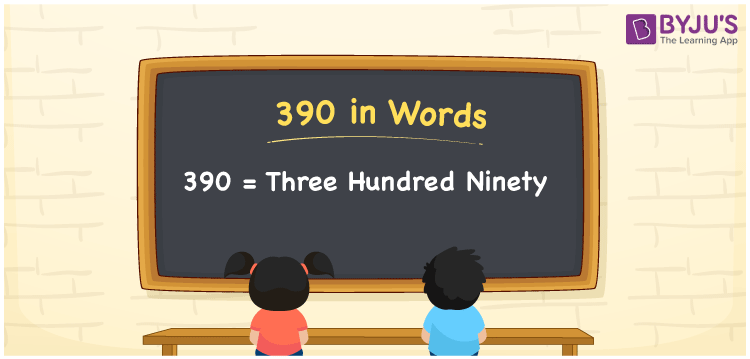# 390 in words

390 in words is written as Three Hundred and Ninety. 390 represents the count or value. The article on Counting Numbers can give you an idea about count or counting. The number 390 is used in expressions that relate to money, days, distance, length, weight and so on. Let us consider an example for 390. “Can we travel around the world in Three Hundred and Ninety Days?” Another example “A large Bear weighs around Three Hundred and Ninety kgs”.

 390 in words Three Hundred and Ninety Three Hundred and Ninety in Numbers 390

## 390 in English Words## How to Write 390 in Words?

We can convert 390 to words using a place value chart. The number 390 has 3 digits, so let’s make a chart that shows the place value up to 3 digits.

 Hundreds Tens Ones 3 9 0

Thus, we can write the expanded form as:

3 × Hundred + 9 × Ten + 0 × One

= 3 × 100 + 9 × 10 + 0 × 1

= 390

= Three Hundred and Ninety.

390 is the natural number that is succeeded by 389 and preceded by 391.

390 in words – Three Hundred and Ninety.

Is 390 an odd number? – No.

Is 390 an even number? – Yes.

Is 390 a perfect square number? – No.

Is 390 a perfect cube number? – No.

Is 390 a prime number? – No.

Is 390 a composite number? – Yes.

## Solved Example

1. Write the number 390 in expanded form

Solution: 3 × 100 + 9 × 10 + 0 × 1

We can write 390 = 300 + 90 + 0

= 3 × 100 + 9 × 10 + 0 × 1

## Frequently Asked Questions on 390 in words

Q1

### How to write 390 in words?

390 in words is written as Three Hundred and Ninety.
Q2

### Is 390 divisible by 2?

Yes. 390 is divisible by 2.
Q3

### Is 390 a perfect square number?

No. 390 is not a perfect square number.